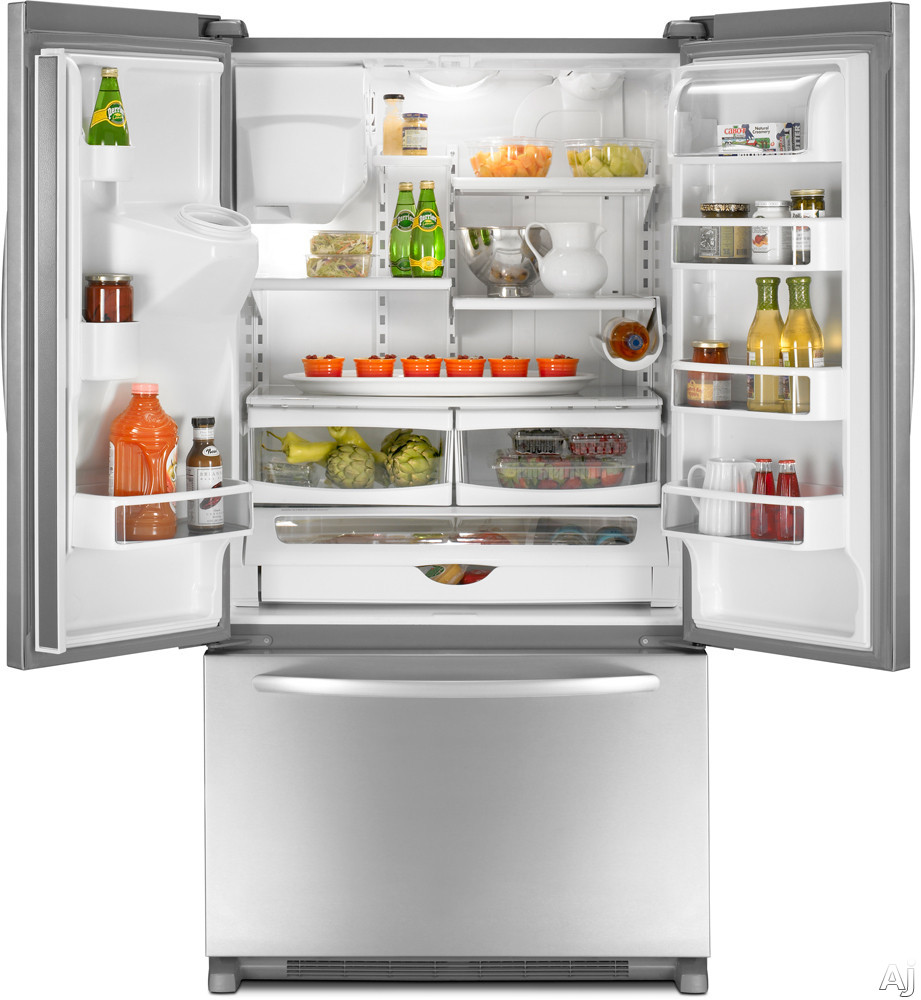# Cool it downYou've recently taken a second job because your leaky refrigerator is driving your energy bill through the roof. As you're wondering where the money for next month's rent is going to come from, you remember a physics class you took long ago.

You decide to model your refrigerator as a simple system: a container with two kinds of mass $m_{\mbox{fridge}}$ and $m_{\mbox{food}}$ with specific heat capacities $c_{\mbox{fridge}}$ and $c_{\mbox{food}}$, respectively. In addition, the refrigerator has a heat pump that can remove heat from the contents of the fridge at a constant rate, $r_{\mbox{pump}}$.

You can either keep the fridge filled with a lot of food $m_{\mbox{fridge}} \approx m_{\mbox{food}}$ or a little food, $m_{\mbox{fridge}} \gg m_{\mbox{food}}$.

Which of these approaches will decrease your fridge's average energy usage the most?

Assumptions

• The refrigerator gains heat by convective heat transfer.
• The pump comes on when the refrigerator reaches the temperature $\Delta T > 0^{\circ} \mbox{ C}$, i.e. $\Delta T = 5^{\circ} \mbox{ C}$, then removes heat until the fridge is at $0^{\circ} \mbox{ C}$ . (Important)
• Both fridges start at $0^{\circ} \mbox{ C}$ at time zero, and we start averaging energy usage from there.
• You've got to cool it down.
×